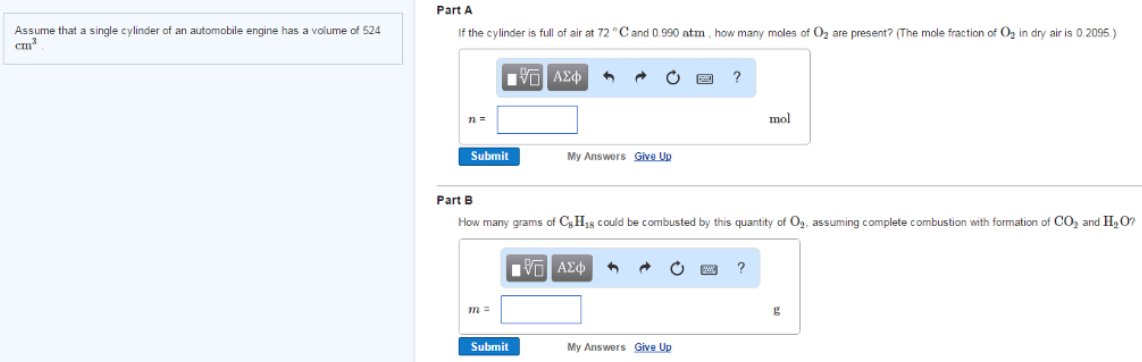# Problem: Assume that a single cylinder of an automobile engine has a volume of 524 cm2.Part AIf the cylinder is full of air at 72°C and 0.990 atm, how many moles of O2 are present? (The mole fraction of O2 in dry is 0.2095)Part BHow many grams of C8H18 could be combusted by this quantity of O2, assuming combustion with formation of CO2 and H2O?

###### FREE Expert Solution
82% (463 ratings)###### Problem Details
Assume that a single cylinder of an automobile engine has a volume of 524 cm2.

Part A
If the cylinder is full of air at 72°C and 0.990 atm, how many moles of O2 are present? (The mole fraction of O2 in dry is 0.2095)

Part B
How many grams of C8H18 could be combusted by this quantity of O2, assuming combustion with formation of CO2 and H2O?